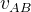## The First Postulate (3)

Suppose in some reference frame, an experiment about Newton's second law of motion is undergoing. What we have to prove, is that when observed from two different reference frames, the formula F=ma works the same way. Since both reference frames are virtual, they have no effect on F and m, so we only have to prove that the accelerations in both reference frames are the same.

In reference frame A, the acceleration is:In reference frame B, the acceleration is:Hereis the reference frame A's speed relative to B. Because both reference frames are inertial,does not change with time, so its change rate with time is 0.

Thus, we have proved that the acceleration in both A and B are really the same.

In the above process, we used the Galileo Transformation:This is a formula we learned in high school physics, which represents the concept of relative motion. So a hidden assumption behind the first postulate is:

All motions are relative.